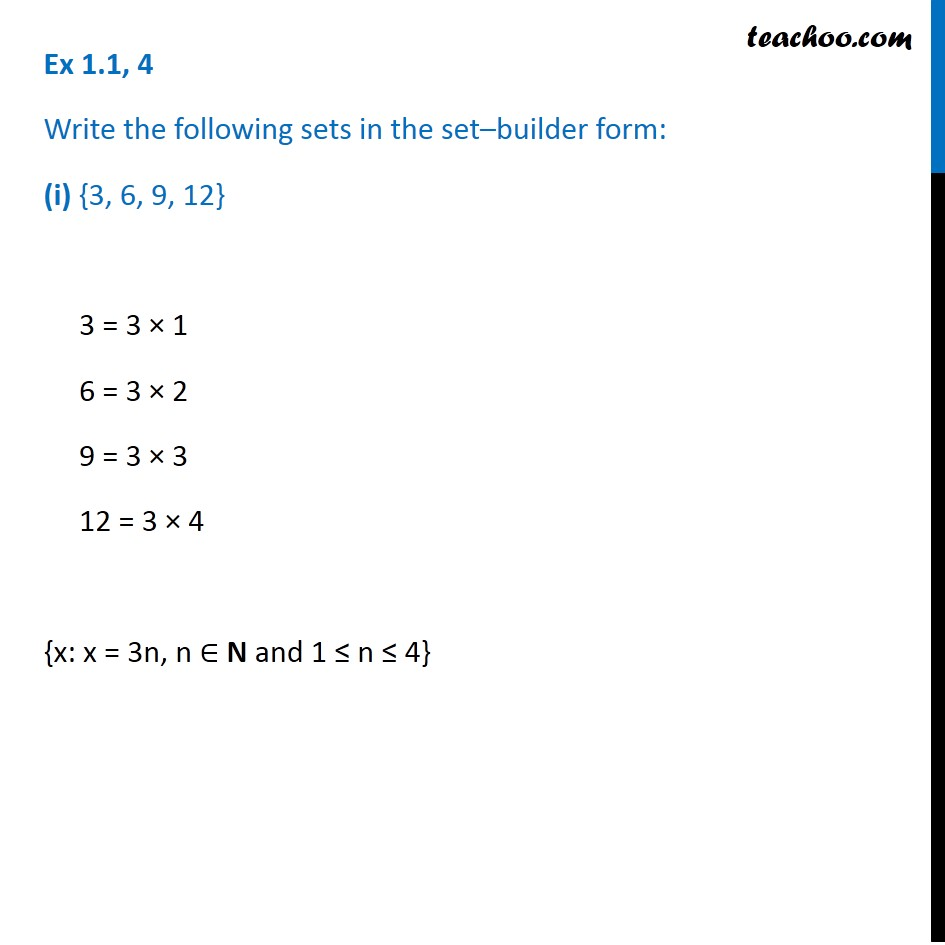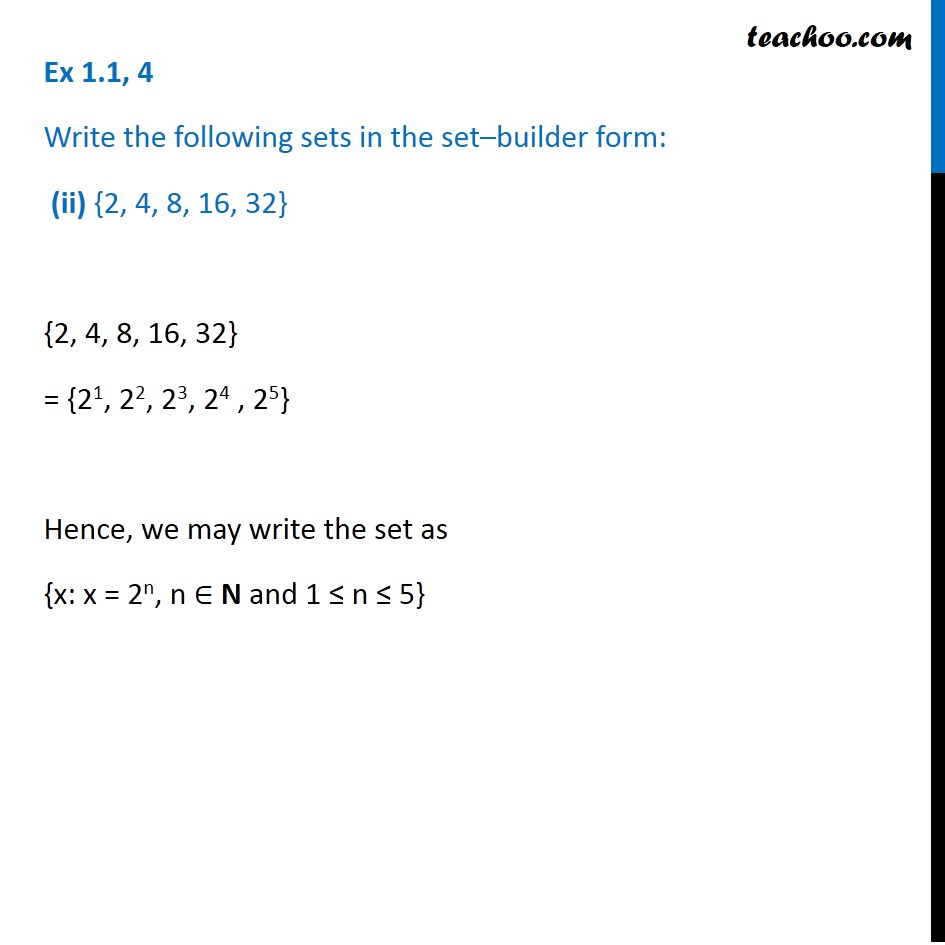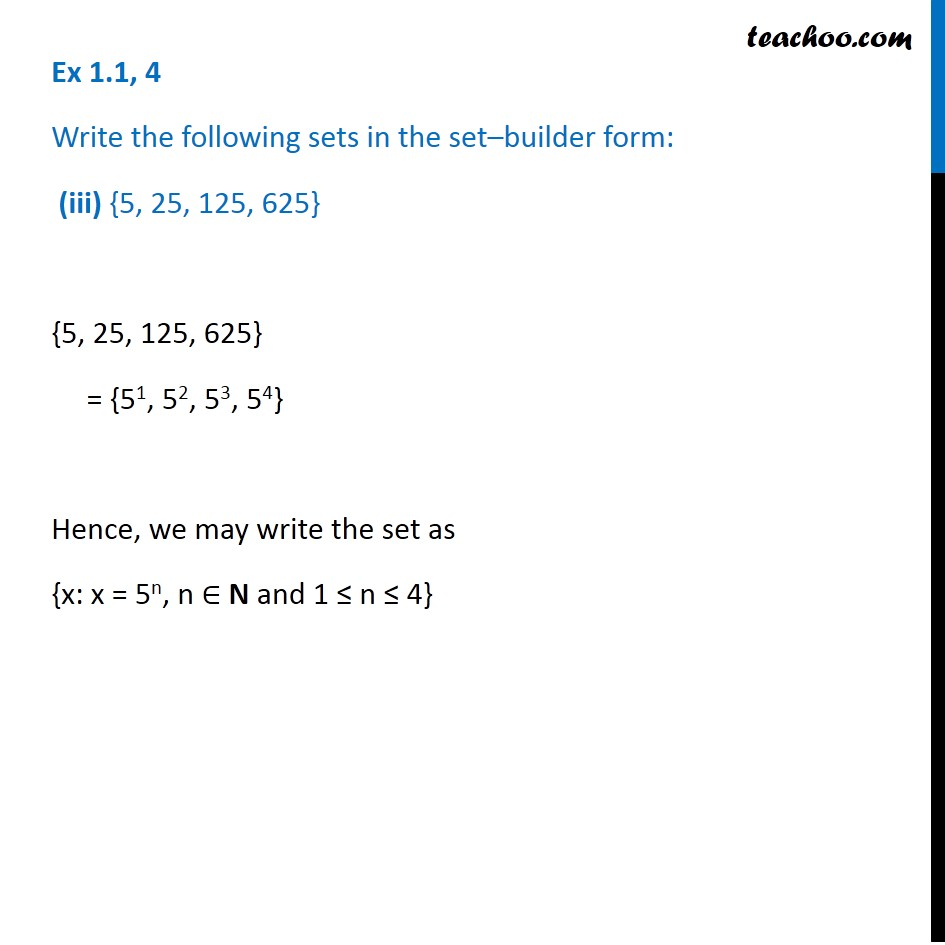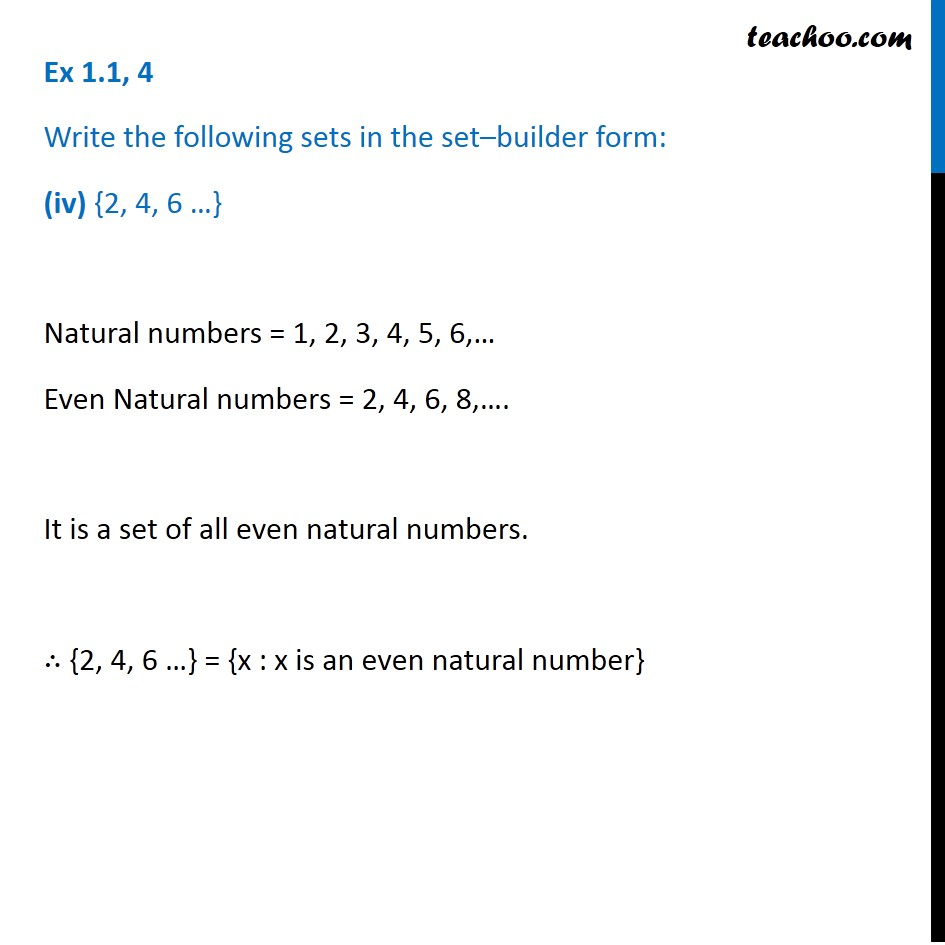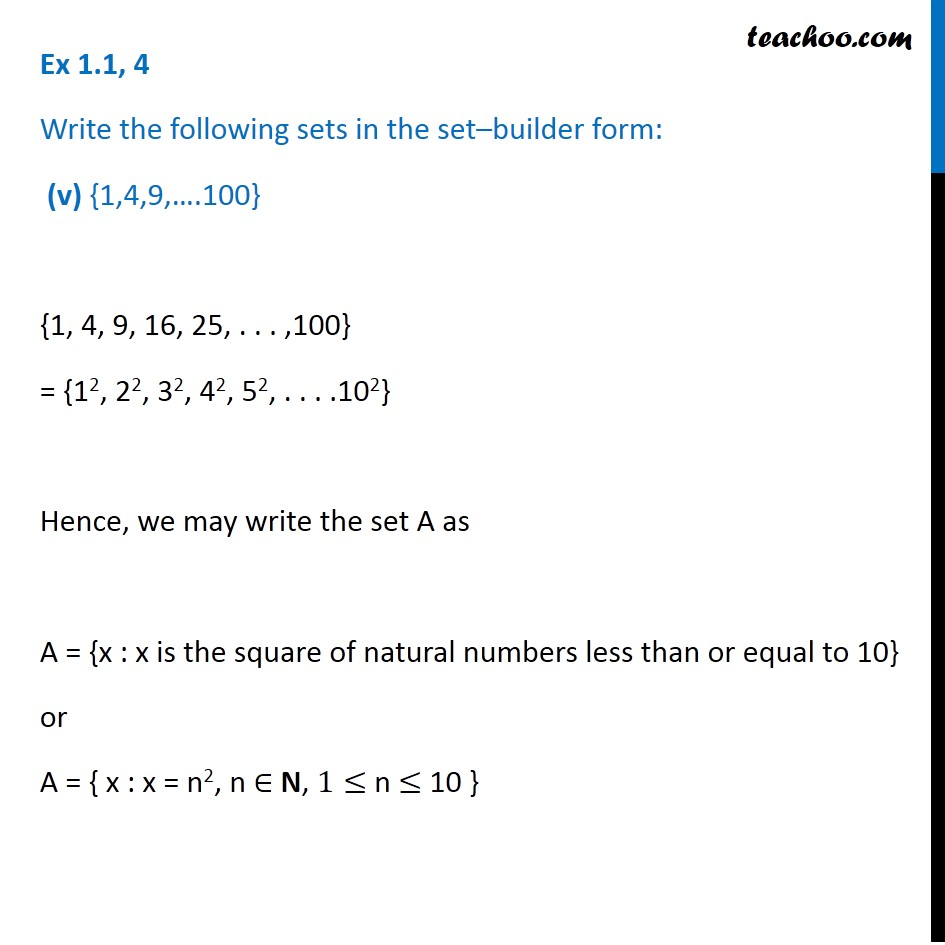1. Chapter 1 Class 11 Sets (Term 1)
2. Serial order wise
3. Ex 1.1

Transcript

Ex 1.1, 4 Write the following sets in the set–builder form: (ii) {2, 4, 8, 16, 32} {2, 4, 8, 16, 32} = {21, 22, 23, 24 , 25} Hence, we may write the set as {x: x = 2n, n ∈ N and 1 ≤ n ≤ 5} Ex 1.1, 4 Write the following sets in the set–builder form: (iii) {5, 25, 125, 625} {5, 25, 125, 625} = {51, 52, 53, 54} Hence, we may write the set as {x: x = 5n, n ∈ N and 1 ≤ n ≤ 4} Ex 1.1, 4 Write the following sets in the set–builder form: (iv) {2, 4, 6 …} Natural numbers = 1, 2, 3, 4, 5, 6,… Even Natural numbers = 2, 4, 6, 8,…. It is a set of all even natural numbers. ∴ {2, 4, 6 …} = {x : x is an even natural number} Ex 1.1, 4 Write the following sets in the set–builder form: (v) {1,4,9,….100} {1, 4, 9, 16, 25, . . . ,100} = {12, 22, 32, 42, 52, . . . .102} Hence, we may write the set A as A = {x : x is the square of natural numbers less than or equal to 10} or A = { x : x = n2, n ∈ N, 1≤ n ≤ 10 }

Ex 1.1